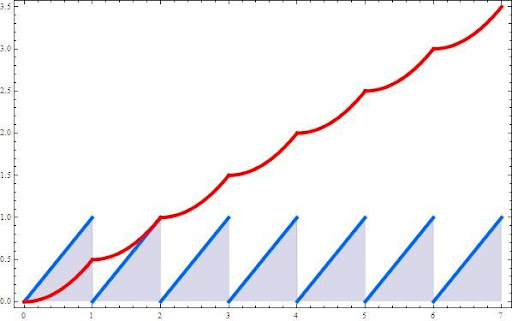## Sunday, 22 March 2009

### INTEGRATING ROUNDING FUNCTIONS-(I)Integer Rounding Functions can be found in many Number Theory texts, but I wasn´t able to find something about its integrals.

The following expressions can be derived just from their plots, adding and subtracting areas. They hold if $latex \displaystyle \;x\geq 0$

The Triangular Numbers function is used to get shorter expressions.

$latex \displaystyle T(n)=\frac{n^{2}+n}{2}$

FLOOR FUNCTION DEFINITE INTEGRAL:

$latex \displaystyle \int_0^x \lfloor x \rfloor dx = \left\{{x}\right\}\lfloor x \rfloor +T(\lfloor x \rfloor-1)$

FRACTIONAL PART FUNCTION DEFINITE INTEGRAL:

$latex \displaystyle \int_0^x \left\{{x}\right\} dx = \frac{x}{2}- \left\{{x}\right\} +T( \left\{{x}\right\} )$

$latex \displaystyle \int_0^x \left\{{x}\right\} dx = \frac{x}{2} +T( \left\{{x}\right\}-1 )$

$latex \displaystyle \int_0^x \left\{{x}\right\} dx =\frac{x+ \left\{{x}\right\}^{2}-\left\{{x}\right\}}{2}$

CEILING FUNCTION DEFINITE INTEGRAL:

$latex \displaystyle \int_0^x \lceil x \rceil dx = \lceil x \rceil (x-\lceil x \rceil)+T(\lceil x \rceil)$

All these formulas can be changed using the relations between them:

$latex \displaystyle \left\{{x}\right\}=x- \lfloor x \rfloor$

This topic doesn't finish here it's going to be used on incoming posts.

Archives:

References:

-Štefan Porubský:Retrieved 2009/3/22 from Interactive Information Portal for Algorithmic Mathematics, Institute of Computer Science of the Czech Academy of Sciences, Prague, Czech Republic http://www.cs.cas.cz/portal/AlgoMath/NumberTheory/ArithmeticFunctions/IntegerRoundingFunctions.htm
-Greg Gamble: The University of Western Australia SCHOOL OF MATHEMATICS & STATISTICS The Floor or Integer Part function